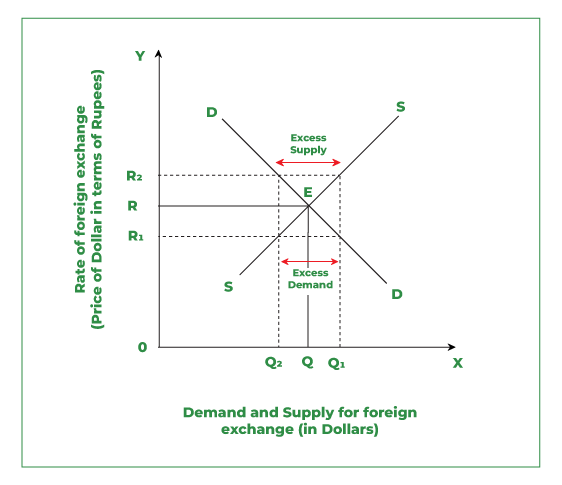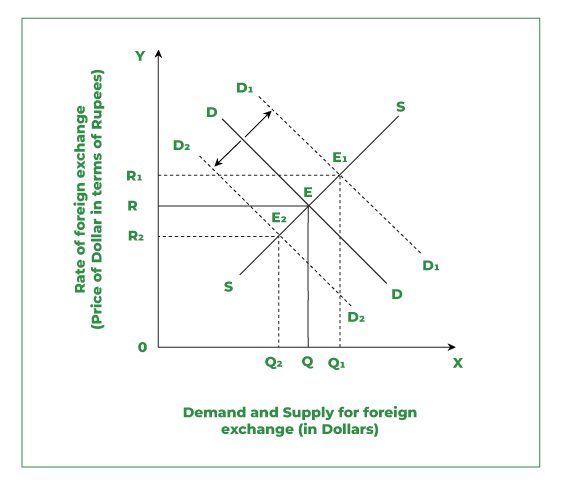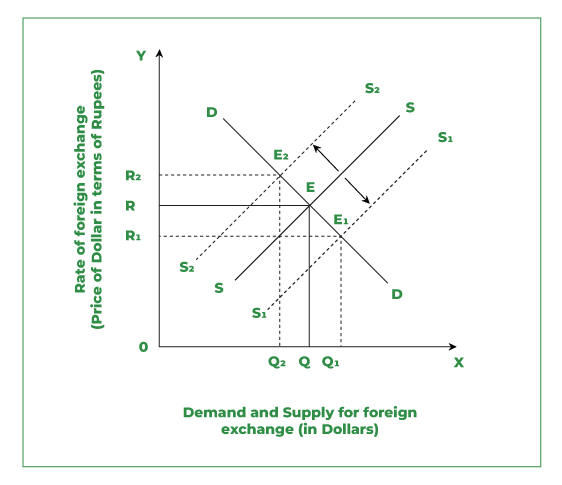Open in App
Not now

# Determination of Exchange Rate

• Last Updated : 27 Oct, 2022

### What is Foreign Exchange?

Foreign exchange refers to foreign currency. For example, for an Indian resident Indian rupee(₹) is a domestic currency that can be used as a medium of exchange in India. But the Indian rupee (₹) can not be used as a medium of exchange outside India. The currency used in other countries is treated as foreign currency for India. Therefore, in the case of international transactions, the domestic currency is converted into foreign currency. For example, if a person visits New York for vacation, he/she can not use the Indian rupee (₹) in New York for economic transactions. The person has to convert the Indian rupee into US Dollars (\$), only then he/she can stay there. For that reason, it is important to know at what price domestic currency can be converted into foreign currency. This price is known as the exchange rate. The market in which domestic currency is traded for others is the “Foreign Exchange Market”.

### Foreign Exchange Rate:

“The rate at which the domestic currency can be exchanged for the foreign currency is known as Foreign Exchange Rate“.

The foreign exchange rate is the price paid in the domestic currency (₹) for buying a unit of foreign currency. It links the currencies of different countries and enables the comparison of international prices. For example, to buy a unit of \$(dollar), if you have to pay ₹60, then the exchange rate is ₹60 per dollar. It can be written in the form; of \$1=₹60.

## Determination of Foreign Exchange Rate

The flexible exchange rate is determined by the forces of demand and supply of foreign exchange in the market. Under this, the equilibrium is established at a point where the quantity demanded is equal to the quantity supplied of foreign exchange, i.e., Demand for foreign exchange is similar to the supply of foreign exchange. This can be shown in Fig 1.Fig 1: Determination of Equilibrium Foreign Exchange Rate

### Observations:

It is evident in the diagram (Fig 1) that the rate of foreign exchange is shown on the Y axis, and the demand and supply of foreign exchange are shown on the X axis.

1. DD is a negatively sloped Demand curve, and SS is a positively sloped Supply curve of foreign exchange that intersects each other at point E.
2. Point E shows the equilibrium between the demand and supply of foreign exchange.
3. Point E corresponds to the OR, which is the equilibrium rate of exchange and OQ, which is the quantity of foreign exchange demanded and supplied.

But now the question arises what if the exchange rate is not the Equilibrium exchange rate?

Case I: At the exchange rate higher than the equilibrium exchange rate, say OR2, there will be excess supply, i.e., Q1Q2. This is so because there is a positive relationship between the price of foreign exchange and the quantity supplied. Thus if the exchange rate rises, the quantity supplied also increases. On the other hand, demand will fall to OQ2, as there is a negative relationship between the price of foreign exchange and the quantity demanded. Thus the excess supply with the fall in demand for foreign exchange will push down the rate of foreign exchange (this indicates that the Indian Rupee will appreciate). It will again lead to an increase in demand from OQ2 to OQ, and a decrease in supply from OQ1 to OQ till it reaches equilibrium E.

Case II: Conversely, at the exchange rate lower than the equilibrium exchange rate says OR1, there will be excess demand, i.e., Q1Q2. This is so because there is a negative relationship between the price of foreign exchange and the quantity demanded. Thus if the exchange rate falls, the quantity demanded increases. On the other hand, supply will fall to OQ2, as there is a positive relationship between the price of foreign exchange and the quantity supplied. Thus the excess demand with an increase in the demand for foreign exchange will push up the rate of foreign exchange (this indicates that the Indian Rupee will depreciate). It will again lead to a decrease in demand from OQ1 to OQ and an increase in supply from OQ2 to OQ till it reaches equilibrium E.

## Changes in Exchange Rate

The foreign exchange rate may rise or fall depending upon the changes in demand and supply of foreign exchange.

### 1. Change in Demand

Change in Demand may be either an “Increase in Demand” or a “Decrease in Demand”

Change in demand is demonstrated in Fig 2. On the Y axis, the Rate of the exchange is shown and on the X axis, the Demand and Supply of foreign exchange are shown. DD is the demand curve and SS supply curve. Both curves (demand and supply) intersect each other at point E. Thus, E is the Equilibrium point. Equilibrium E corresponds to the OR Rate of foreign exchange rate and OQ quantity demanded.Fig 2: Change in Demand

#### Increase in Demand:

The increase in the demand for foreign exchange will shift the demand curve from DD to D1D1. It can be seen in Fig 2 that due to a shift in the demand curve, the supply curve will now meet the demand curve at E1, which will be the new Equilibrium point. Now, at the new equilibrium point, the exchange rate will increase to OR1 with quantity demanded as OQ1. It means that with an increase in demand, the exchange rate will also increase.

#### Decrease in Demand:

The decrease in the demand for foreign exchange will shift the demand curve from DD TO D2D2. It can be seen in Fig 2 that due to a shift in the demand curve, the supply curve will now meet the demand curve at E2, which will be the new Equilibrium point. Now, at the new equilibrium point, the exchange rate will decrease to OR2 with quantity demanded as OQ2. It means that with a decrease in demand, the exchange rate will also decrease.

### Change in Supply

Change in Supply may be either an “Increase in Supply” or a “Decrease in Supply”.

Change in supply is demonstrated in Fig 3. On the Y axis, the Rate of exchange is shown and on the X axis, the Demand and Supply of foreign exchange are shown. DD is the demand curve and SS supply curve. Both curves (demand and supply) intersect each other at point E. Thus E is the Equilibrium point. Equilibrium E corresponds to the OR Rate of foreign exchange rate and OQ quantity supplied.Fig 3: Changes in Supply

#### 1. Increase in Supply:

The increase in the supply of foreign exchange will shift the supply curve from SS to S1S1. It can be seen in Fig 3 that due to a shift in the supply curve, the demand curve will now meet the supply curve at E1, which is the new Equilibrium point. Now, at the new equilibrium point, the exchange rate will decrease to OR1 with quantity demanded as OQ1.. It means that with an increase in supply, the exchange rate will decrease.

#### 2. Decrease in Supply:

The decrease in the supply of foreign exchange will shift the supply curve from SS to S2S2. It can be seen in Fig 3 that due to a shift in the supply curve, the demand curve will now meet the supply curve at E2, which is the new Equilibrium point. Now, at the new equilibrium point, the exchange rate will increase to OR2 with quantity demanded as OQ2. It means that with a decrease in supply, the exchange rate will increase.

My Personal Notes arrow_drop_up
Related Articles